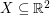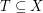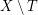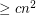# empty triangle

## Monochromatic empty triangles ★★★

Author(s):

Ifis a finite set of points which is 2-colored, an empty triangle is a setwithso that the convex hull ofis disjoint from. We say thatis monochromatic if all points inare the same color.

Conjecture   There exists a fixed constantwith the following property. Ifis a set ofpoints in general position which is 2-colored, then it hasmonochromatic empty triangles.

Keywords: empty triangle; general position; ramsey theory